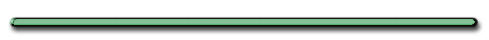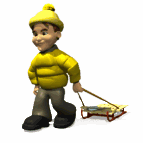HomeWhat crushed this can?

When the can is immersed in the water, the pressure inside the can is lower than the pressure outside

Review

Click on the button and play ...

 One of these things is not like the other!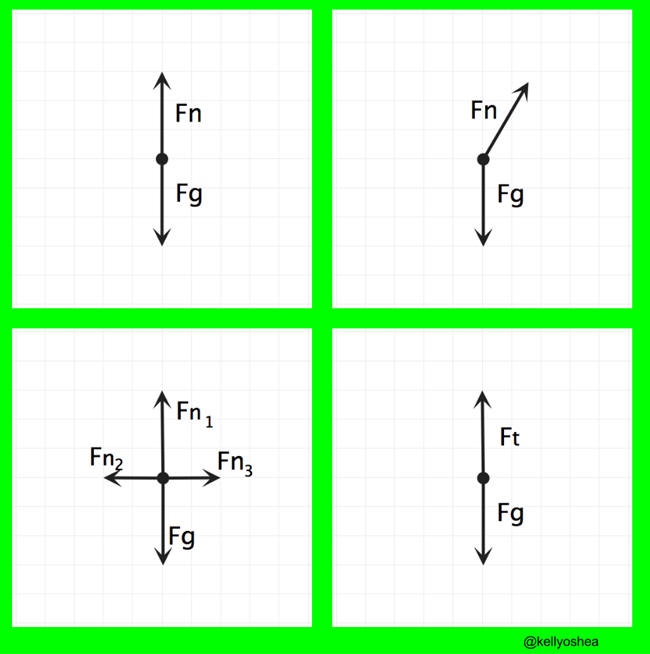The idea for this game and graphic above come from a great high school physics teacher named Kelly O'Shea.HaeLim (Emily) Wee , Class of 2017

Video Instruction

showmethephysics.comForce on refrigerator acts in 2 directions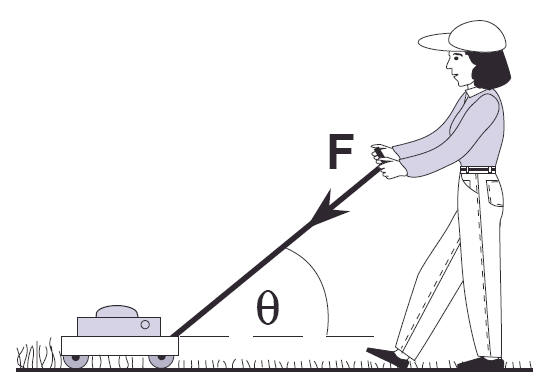Force acts in 2 directions

H. Resolution of Forces - Breaking down a single vector into 2 or more vectors

Show Me The Physics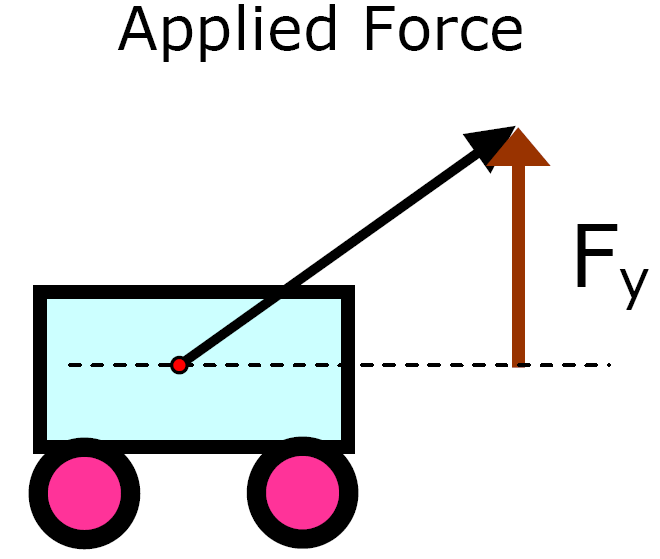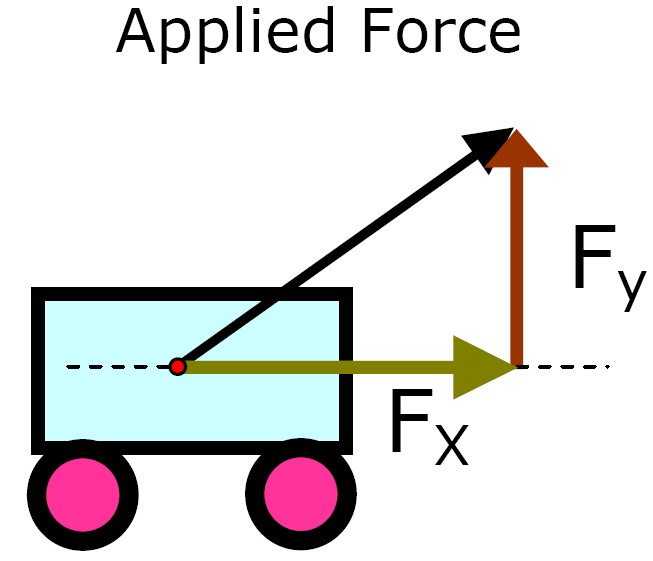Which component is responsible
for making this cart move?

Only horizontal part (Fx)
of applied force

If the angle were increased, how would it change the size of the:

Vertical component?

Increase

Horizontal component?

Decrease

Video Instruction

showmethephysics.com

Ex 1) A car is pulled with a force of 60. N at angle of 37° from the horizontal. Find the vertical and horizontal components of the applied force

Scale: 1 cm = 10 N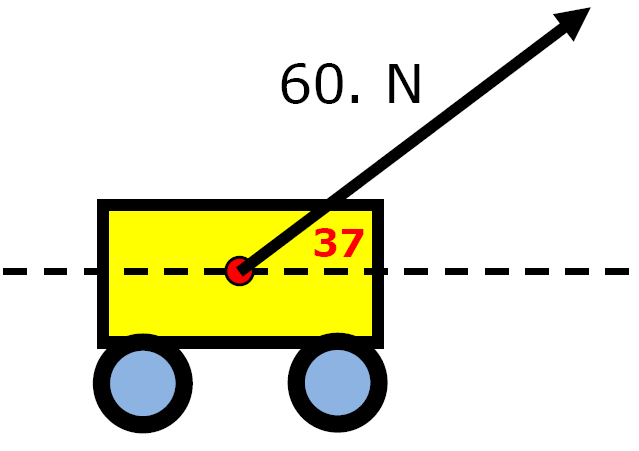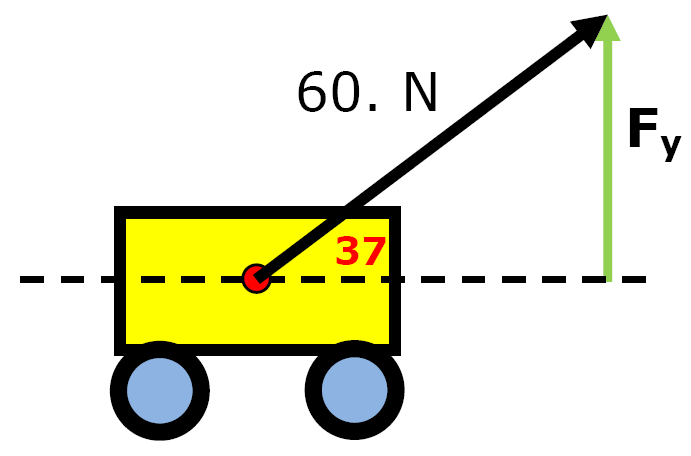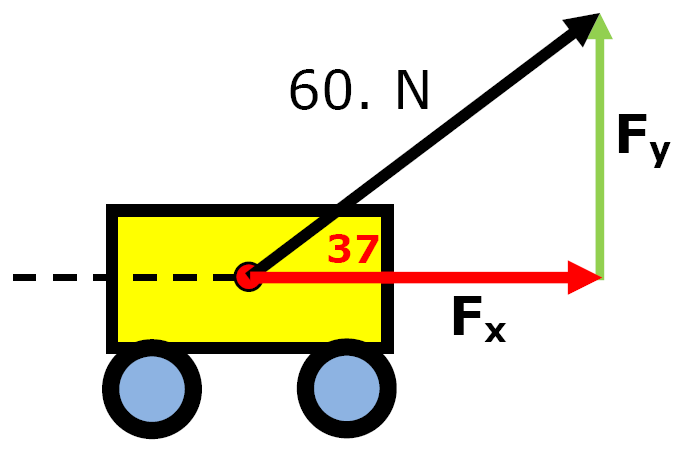Using Trigonometry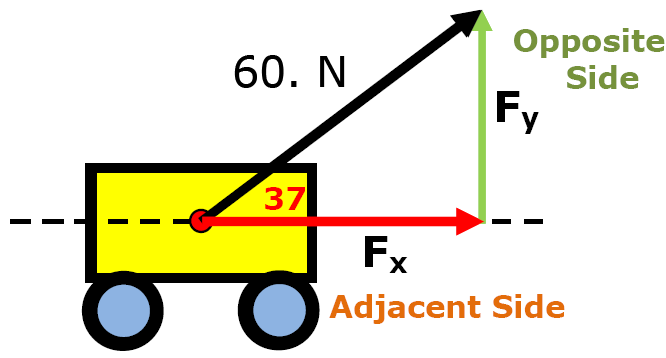SinӨ = Opp = Fy Hyp F

Cross Multiply

 Fy = FsinӨ

 Fx = FcosӨ

"One size fits all"Fy = 60. Nsin37°

Fy = 36 N Up

Fx = 60. Ncos37°

Fx = 48 N Right

Check  a2 + b2 = c2

36 N2 + 48 N2= 60. N2

Ex 2) Weight of Cart = 80 N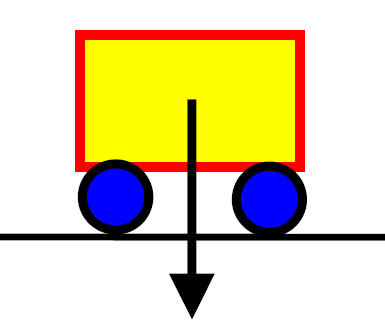Horizontal Component
of Weight Force?

O N

Vertical Component
of Weight Force?

80. N

Horizontal Component

0 NVertical Component

80 N down

Ex 3) A 50. N force at an angle of 80° has its' angle changed to 10.°.

How will the vertical and horizontal components change?

Angle & Components - Flash

Show me The Physics Website

Vertical component - smaller
Horizontal component - largerRanking Task Rank the Vertical Components from least to greatest.Explain your answers

Ex 4) Parallel & perpendicular components

Show Me The PhysicsF = 10. N (Weight)F = 10. N (Weight)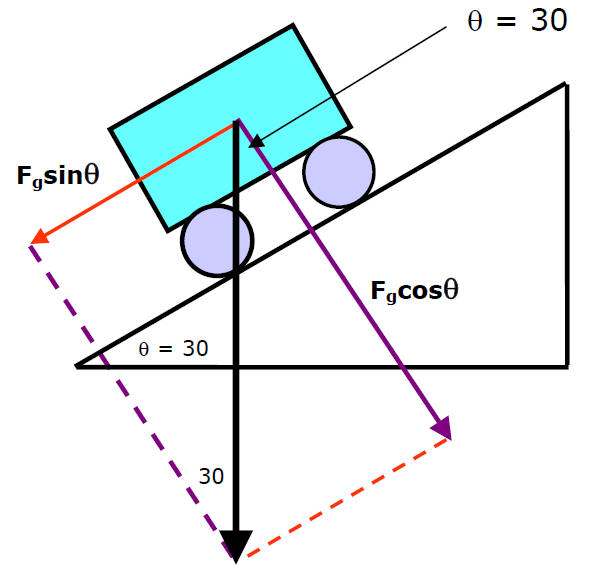Find the force holding cart on ramp and force that acts down

Force (down ramp) = Fapplied sinӨ = 5.0 N
Force
(on ramp) = Fapplied cosӨ = 8.7 N

 Ranking Task Rank the parallel component from greatest to smallestRank the perpendicular component from greatest to smallest

Finding a horizontal component

Box on a Ramp

Show Me The PhysicsDynamics**AP - **